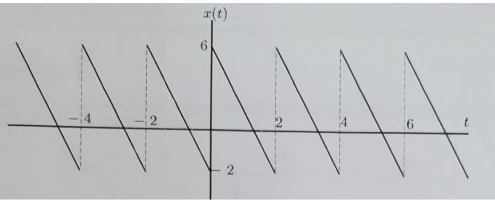# Fourier series - where is f(t) discontinuous

#### Randyyy

##### Junior Member
The function f is periodic with [imath]T=2\pi[/imath] and is given as f(t)=t where [imath]-\pi \leq t < \pi[/imath]. Is the function odd, even or neither? Also, what points are f(t) discontinuous?

My attempt:

f(-t)=-t, hence f is an odd function. I then set out to find the Fourier series corresponding to f(t) which gives me:

$\sum_{n=1}^\infty \dfrac{2}{n}(-1)^{n+1}$
which is correct, but I don't get how f(t) can be discontinuous? I get that if n=0 in the series above we get issues but other than that I have no clue how f is discontinuous at any points since it is a linear function. I probably need to utilize the fact that I know the period of f(t).

#### Zermelo

##### Junior Member
Hi there,
Your class probably teaches the Dirichlet’s conditions for Fourier series. One of those conditions is that the function must be periodic. If you take a look at the function f(x) = x, it isn’t periodic! Just look at the graph, it’s a line splitting the first and third quadrant. In order to use this method, you need to make your function periodic, so the graph will look similar to this:Except that this function is 2-periodic, and is not the function f(x) = x
Now you should see where the points of discontonuity are!